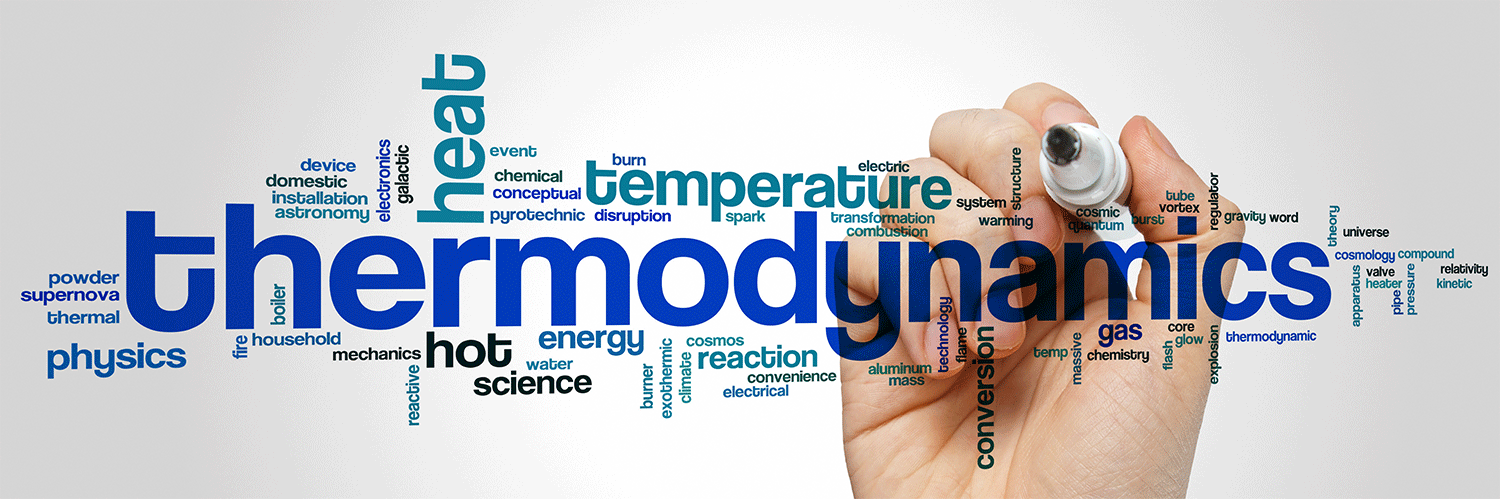# Cryogenic Thermodynamics

For those in the Cryogenic field, thermodynamics are a critical element that affect the delivery of cryogenic liquids and keep things… well, cryogenic.It is recommended to review the basic laws of Thermodynamics that apply to cryogenic liquids first.  There are three basic laws of thermodynamics:

## First Law of Thermodynamics

Energy can be exchanged between the system of interest and its surroundings.  However, the total energy of the system plus the surroundings is constant.  That’s the First Law of Thermodynamics.  The First Law is also stated as energy is conserved.

This is an empirical law, which means that we know that energy is conserved because of many repeated experiments by scientists.  It’s been observed that you can’t get any more energy out of a system than you put into it.  James Prescott Joule did a famous experiment which demonstrated the conservation of energy and showed that heat and work were both of the same nature: energy.  His system of interest was water in a thermally insulated container.  In this container was also a paddle, which was connected to the outside world (surroundings) and connected to weights on a string.  Joule measured the work done by the paddle wheel and he also measured the heat created by the wheel turning in the water.

Significantly, Joule found that the amount of energy done as work was converted exactly to heat.  Energy was changed from one form to another (work to heat); however, no net change of energy in the system plus the surroundings occurred.  Energy is conserved.

## Second Law of Thermodynamics

The second law is concerned with entropy (S), which is a measure of disorder.  The second law says that the entropy of the universe increases.  An increase in disorder (overall) is therefore spontaneous.  If the volume and energy of a system are constant, then every change to the system increases the entropy.  If volume or energy change, then the entropy of the system can actually decrease.  However, the entropy of the universe does not decrease.  The molecules in one’s body exist in great order; this only happens because the entropy of the rest of the universe is increased to a greater amount than the entropy of the body is decreased.

The laws of thermodynamics were determined empirically (by experiment).  They are generalizations of repeated scientific experiments.  The second law is a generalization of experiments dealing with entropy — it is that the S of the system plus the S of the surroundings is equal to or greater then 0.  Entropy is not conserved, like energy.

## Third Law of Thermodynamics

The third law of thermodynamics states that the entropy of a pure perfect crystal is 0 at 0K: S(0K) = 0.  At 0K the atoms in a pure perfect crystal are aligned perfectly and do not move.  Moreover, there is no entropy of mixing since the crystal is pure.  For a mixed crystal containing the atomic or molecular species A and B, there are many possible arrangements of A and B and there is therefore entropy associated with the arrangement of the atoms/molecules.